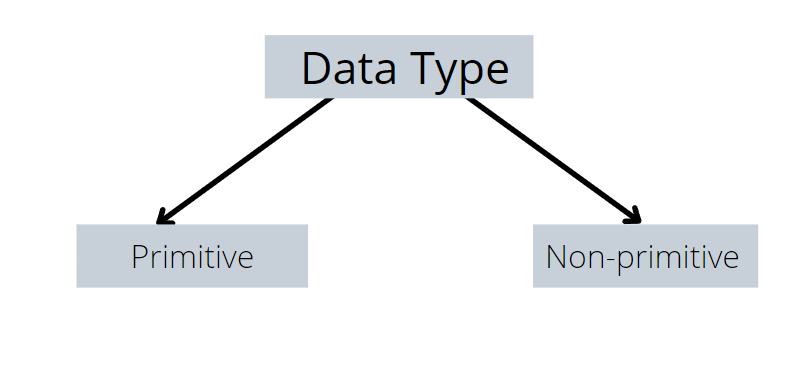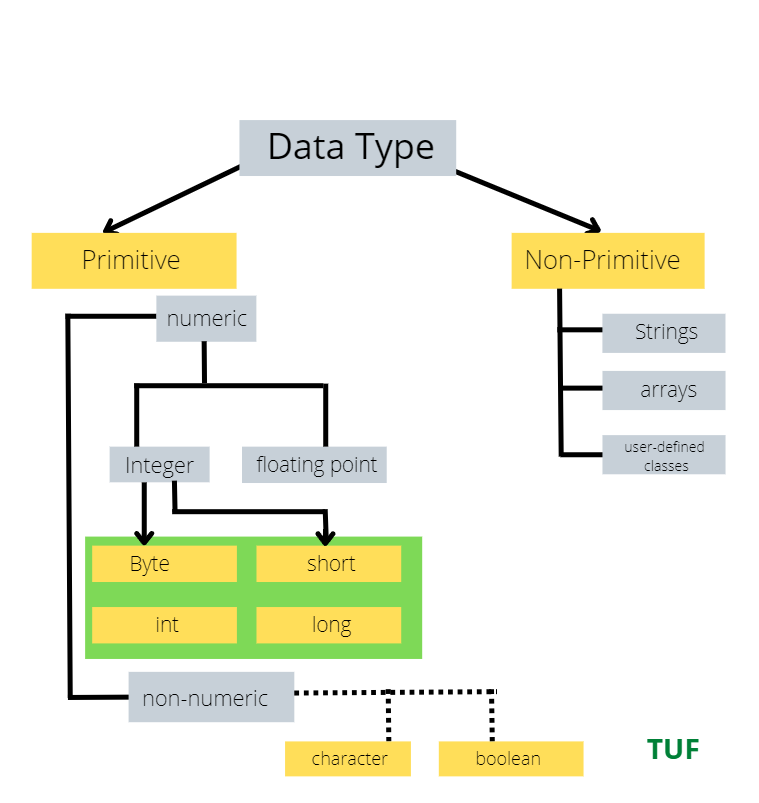# Java Data Types

In this tutorial, we will learn about Data Types in Java.

### What is Data Type?

Data types define the size and value a variable can hold. There are majorly 2 types of data types in Java.Java is a statically-typed language. This means that all variables must be declared before they can be used.

### Types of Data Types:• Primitive -There are 8 primitive types available in Java: byte, short, int, long, char, float, double, and boolean.
• Non-primitive – Non-primitive data types are classes, interfaces and arrays, and Strings.### Primitive Data Types:

#### Short type:

• This data type can hold integral numbers from -32,768 to 32,767.
• can have a size of 2 bytes or 16 bits.
• The default value is 0.

Example:

## Java Code

``````class Main {
public static void main(String[] args) {

short temperature;
temperature = 900;
System.out.println(temperature);  // prints 900
}}
``````

#### Byte type:

• This is an 8 bit or 1 byte signed data type which can store integers lying between -125 and 172.
• The default value is 0.

Example:

## Java Code

``````class Main {
public static void main(String[] args) {

byte range;
range = 29;
System.out.println(range);    // prints 29
}}
``````

### Int type :

• This data type can store values up to 4 bytes or 32 bits.It can store whole numbers between -2,147,483,648 and 2,147,483,647.
• This data type is usually used for storing numeric values in programs.

Example:

## Java Code

``````class Main {
public static void main(String[] args) {
int a = -6510300;
System.out.println(a);  // print -4250000
}
}
``````

#### Long type:

• This data type can have the size of 8 bytes or 64 bits or numbers from  -263 to 263-1.
• The default value is 0.

Example:

## Java Code

``````class LongExample {
public static void main(String[] args) {

long range = -91232200000L;
System.out.println(range);    // prints -91232200000L
}}``````

#### Double type:

• This data type can store values or numbers from 1.7e−308 to 1.7e+308, ending with a ‘d’ character,
• Default value is 0.0d.

Example:

## Java Code

``````class Main {
public static void main(String[] args) {

double number = -13.7;
System.out.println(number);  // prints -13.7
}
}
``````

#### Float type:

• This can store fractional numbers with 4 bytes of memory.
• The float data type can have numbers from 3.4e−03 to 3.4e+038, where 3.4e−038 is the smallest positive value of float.
• Default value is 0.0f.

Example:

## Java Code

``````class Main {
public static void main(String[] args) {
float num = -12.6f;
System.out.println(num);  // prints -12.6
}
}
``````

#### Char type:

• This data type can be used to store a single character value, for example, ‘e’, ‘@’, enclosed between single quotes.
• Default value  ‘\u0000’.

Example:

## Java Code

``````class Main {
public static void main(String[] args) {

char characters = 'a';
System.out.println(characters);  // prints a
}
}
``````

#### Boolean type:

• The boolean data type has only two possible values i.e True or False,
• The default value is False

Example:

## Java Code

``````class Main {
public static void main(String[] args) {

boolean flag = true;
System.out.println(flag);    // prints true
}
}
``````

### Non-Primitive Data Types:

• Unlike primitive data types, these are not predefined.
• These are user-defined data types created by programmers. These data types are used to store multiple values.

#### Strings in java:

• A string represents a sequence of characters, for example, “Take You Forward!”
`Example : String s = "Striver";`

#### Arrays In java:

• An array is a data type that can store multiple homogenous variables i.e., variables of the same type in a sequence.
• In Java, all arrays are dynamically allocated and each has an index beginning from 0.
```Example : int[ ] arr = {2,4,7};
```

### Class

• A class defines the attributes and behavior of an object.
• It consists of member variables and methods.

Example:

## Java Code

``````public class Main {
int x = 29;

public static void main(String[] args) {
Main myObj = new Main();
System.out.println(myObj.x); // prints 29
}
}
``````

#### Interface:

• An interface is declared like a class but the only difference is that it contains only final variables and method declarations.
• It is a fully abstract class, used to achieve abstraction.
• An interface cannot contain a constructor and that’s why it can not be used to create objects.

Special thanks to Amisha Kumari for contributing to this article on takeUforward. If you also wish to share your knowledge with the takeUforward fam, please check out this article